Normal view MARC view ISBD view

Microsoft Excel 2019 bible / by Michael Alexander, Dick Kusleika, and John Walkenbach

Publisher: Indianapolis, I.N. : Wiley, 2019Description: xlv, 1074 p. ; electronic resource.ISBN: 9781119514787.Online resources: Download as an eBook
Contents:
GETTING STARTED WITH EXCEL: Introducing Excel -- Entering and editing worksheet data -- Performing basic worksheet operations -- Working with Excel ranges and tables -- Formatting worksheets -- Understanding Excel files and templates -- Printing your work -- Customizing the Excel user interface. WORKING WITH FORMULAS AND FUNCTIONS: Introducing formulas and functions -- Using formulas for common mathematical operations -- Using formulas to manipulate text -- Using formulas with dates and times -- Using formulas for conditional analysis -- Using formulas for matching and lookups -- Using formulas for financial analysis -- Using formulas for statistical analysis -- Using formulas with tables and conditional formatting -- Understanding and using array formulas -- Making your formulas error-free. CREATING CHARTS AND OTHER VISUALIZATIONS: Getting started with Excel charts -- Using advanced charting techniques -- Creating sparkline graphics -- Visualizing with custom number formats and shapes -- Implementing Excel dashboarding best practices. MANAGING AND ANALYZING DATA: Importing and cleaning data -- Using data validation -- Creating and using worksheet outlines -- Linking and consolidating worksheets -- Introducing pivottables -- Analyzing data with pivottables -- Performing spreadsheet what-if analysis -- Analyzing data using goal seeking and solver -- Analyzing data with the analysis toolpak -- Protecting your work. UNDERSTANDING POWER PIVOT AND POWER QUERY: Introducing power pivot -- Working directly with the internal data model -- Adding formulas to power pivot -- Introducing power query -- Transforming data with power query -- Making queries work together -- Enhancing power query productivity. AUTOMATING EXCEL: Introducing visual basic for applications -- Creating custom worksheet functions -- Creating userforms -- Using userform controls in a worksheet -- Working with Excel events -- Seeing some VBA examples -- Creating custom Excel add-ins.
Summary: Provides a complete guide to Excel 2019 for beginner, intermediate and advanced users. Discusses the basics of using worksheets, formulas, functions and charts. Describes how to analyse data, create pivot tables, and take advantage of Excel tools such as Sparklines and VBA macros. Written for the desktop version of Excel 2019 and can also be applied to the web and tablet versions. Not applicable to Excel for Mac.
Item type Home library Call number URL Status Notes Date due Item reservesEBook
EBL Ebook (Browse shelf) Link to resource eBook Available as an ebook here
Total holds: 0
Browsing International Shelves Close shelf browser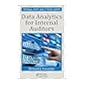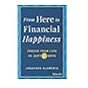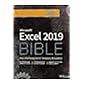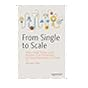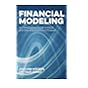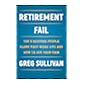EBL Ebook Data analytics for internal auditors EBL Ebook 101 ways to save money on your tax - legally! 2018-2019 : EBL Ebook From here to financial happiness : EBL Ebook Microsoft Excel 2019 bible EBL Ebook From single to scale : EBL Ebook Financial modeling : EBL Ebook Retirement fail :

GETTING STARTED WITH EXCEL: Introducing Excel -- Entering and editing worksheet data -- Performing basic worksheet operations -- Working with Excel ranges and tables -- Formatting worksheets -- Understanding Excel files and templates -- Printing your work -- Customizing the Excel user interface. WORKING WITH FORMULAS AND FUNCTIONS: Introducing formulas and functions -- Using formulas for common mathematical operations -- Using formulas to manipulate text -- Using formulas with dates and times -- Using formulas for conditional analysis -- Using formulas for matching and lookups -- Using formulas for financial analysis -- Using formulas for statistical analysis -- Using formulas with tables and conditional formatting -- Understanding and using array formulas -- Making your formulas error-free. CREATING CHARTS AND OTHER VISUALIZATIONS: Getting started with Excel charts -- Using advanced charting techniques -- Creating sparkline graphics -- Visualizing with custom number formats and shapes -- Implementing Excel dashboarding best practices. MANAGING AND ANALYZING DATA: Importing and cleaning data -- Using data validation -- Creating and using worksheet outlines -- Linking and consolidating worksheets -- Introducing pivottables -- Analyzing data with pivottables -- Performing spreadsheet what-if analysis -- Analyzing data using goal seeking and solver -- Analyzing data with the analysis toolpak -- Protecting your work. UNDERSTANDING POWER PIVOT AND POWER QUERY: Introducing power pivot -- Working directly with the internal data model -- Adding formulas to power pivot -- Introducing power query -- Transforming data with power query -- Making queries work together -- Enhancing power query productivity. AUTOMATING EXCEL: Introducing visual basic for applications -- Creating custom worksheet functions -- Creating userforms -- Using userform controls in a worksheet -- Working with Excel events -- Seeing some VBA examples -- Creating custom Excel add-ins.

Provides a complete guide to Excel 2019 for beginner, intermediate and advanced users. Discusses the basics of using worksheets, formulas, functions and charts. Describes how to analyse data, create pivot tables, and take advantage of Excel tools such as Sparklines and VBA macros. Written for the desktop version of Excel 2019 and can also be applied to the web and tablet versions. Not applicable to Excel for Mac.

© 2017 Chartered Accountants Australia and New Zealand ABN 50 084 642 571 (CA ANZ).
Formed in Australia. Members of CA ANZ are not liable for the debts and liabilities of CA ANZ